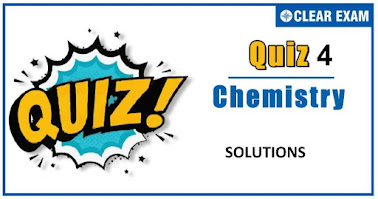## [LATEST]\$type=sticky\$show=home\$rm=0\$va=0\$count=4\$va=0

Q1. 25 mL of a solution of barium hydroxide on titration with 0.1 molar solution of hydrochloric acid gave a titre value of 35 mL. The molarity of barium hydroxide solution was
•  g/100 cc
•  g/L
•  Mg/100 cc
•  Ppm
Solution
The concentration is expressed in parts per million (ppm) when one part of solute is dissolved in one million parts of solvent

Q2.1.0 g of a non-electrolyte solute (molar mass 250 g mol^(-1)) was dissolved in 51.2 g of benzene. If the freezing point depression constant of benzene is 5.12 K kg mol^(-1), the lowering in freezing point will be ::
•  0.5 K
•  0.4 K
•  0.2 K
•  0.3
Solution
∆T=(1000 ×K_f×w)/(m×W) =(1000 ×5.12×1)/(250 ×51.2) = 0.4 K

Q3.  The mole fraction of the solute in one modal aqueous solution is
•   0.048
•  0.036
•  0.027
•  0.018
Solution
w=1000 g(H_2 O);n=1 mol N=W/M=1000/18=55.55 X_solute=n/(n+N)+1/(1+55.55) =0.018

Q4. The osmatic pressure of a 5% (wt./vol) solution of cane sugar at 150℃ is
•  3.078 atm
•  4.078 atm
•  2.45 atm
•  5.078 atm
Solution
C=5/342×1/100×1000=50/342 mol/L π=50/342×0.082×423=5.07 atm

Q5.Conc〖 H〗_2 SO_4 has a density of 1.98 g/mL and is 98% H_2 SO_4 by weight. Its normality is
•  39.6 N
•  49.6 N
•  29.6 N
•  19.6 N
Solution
Strength of H_2 SO_4=98×19.8 g/L S=eq.wt.×N N=S/(eq.wt.)=(98×19.8)/49=39.6

Q6. After adding a solute freezing point of solution decreases to -0.186. Calculate ∆T_b if k_f=1.86 and k_b=0.512
•  1.86
•  0.0512
• 0.0186
•  0.512
Solution

Q7.The freezing point (in °C)of a solution containing 0.1 g of K_3 [Fe(CN)_6] (mol.wt.329) in 100 g of water is : (K_f=1.86 K kg mol^(-1))
•  -1.2 ×〖10〗^(-2)
•  -5.7 ×〖10〗^(-3)
•  -2.3 ×〖10〗^(-2)
•  -5.7 ×〖10〗^(-2)
Solution
Q8.A mixture of ethyl alcohol and propyl alcohol has a vapour pressure of 290 mm at 300 K. the vapour pressure of propyl alcohol is 200 mm. If the mole fraction of ethyl alcohol is 0.6, its vapour pressure (in mm) at the same temperature will be
•  300
•  350
•  700
•  360
Solution

Q9.The values of observed and calculated molecular weights of silver nitrate are 92.64 and 170 respectively. The degree of dissociation of silver nitrate is :
•  60%
•  60.23%
•  46.7%
•  83.5%
Solution
Q10. At 40 ͦC the vapour pressure in torr, of methyl alcohol-ethyl alcohol solutions is represented by the equation. P=119 X_A+135; where X_A is mole-fraction of methyl alcohol, then the value of lim┬(X_A⟶1)⁡〖P_A/X_A 〗is :
•  135 torr
•  254 torr
•  119 torr
• 140 torr
Solution#### Written by: AUTHORNAME

AUTHORDESCRIPTION## Want to know more

Please fill in the details below:

## Latest NEET Articles\$type=three\$c=3\$author=hide\$comment=hide\$rm=hide\$date=hide\$snippet=hide

Name

ltr
item
BEST NEET COACHING CENTER | BEST IIT JEE COACHING INSTITUTE | BEST NEET, IIT JEE COACHING INSTITUTE: SOLUTIONS QUIZ-4
SOLUTIONS QUIZ-4
https://1.bp.blogspot.com/-95CNrVATFHc/X57yCi6mOJI/AAAAAAAAIjc/W3UhQ8NjpmcDOywAo47eYTfPVRBGl8VsgCLcBGAsYHQ/w378-h223/Quiz%2BImage%2BTemplate%2B%252833%2529.jpg
https://1.bp.blogspot.com/-95CNrVATFHc/X57yCi6mOJI/AAAAAAAAIjc/W3UhQ8NjpmcDOywAo47eYTfPVRBGl8VsgCLcBGAsYHQ/s72-w378-c-h223/Quiz%2BImage%2BTemplate%2B%252833%2529.jpg
BEST NEET COACHING CENTER | BEST IIT JEE COACHING INSTITUTE | BEST NEET, IIT JEE COACHING INSTITUTE
https://www.cleariitmedical.com/2020/11/solutions-quiz-4.html
https://www.cleariitmedical.com/
https://www.cleariitmedical.com/
https://www.cleariitmedical.com/2020/11/solutions-quiz-4.html
true
7783647550433378923
UTF-8